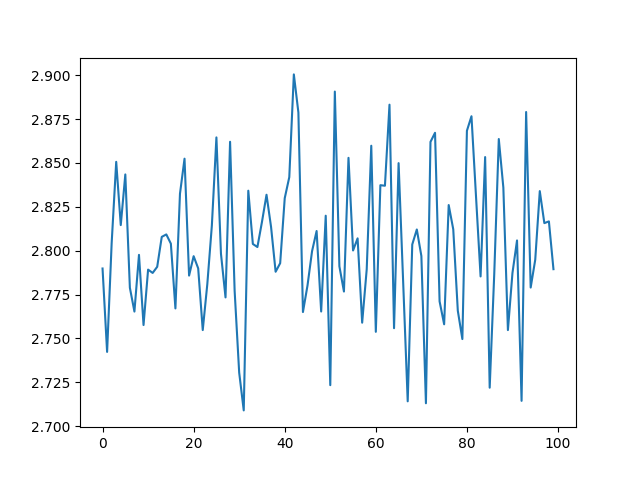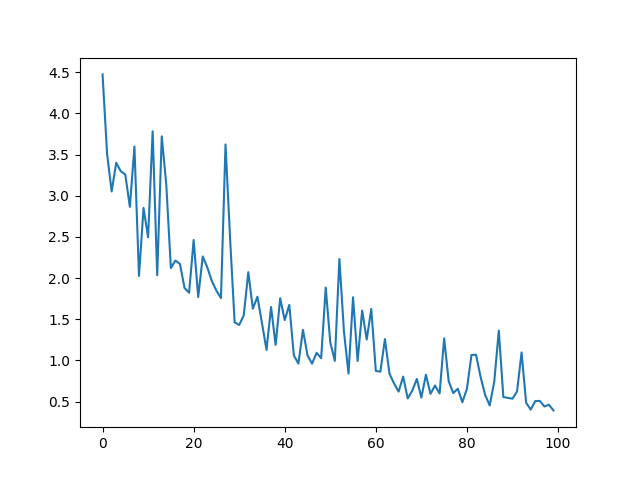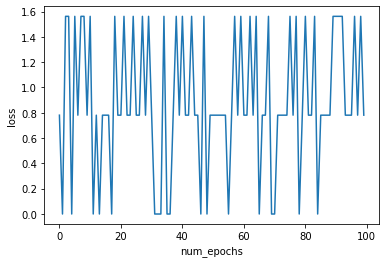# LSTM training loss does not decrease

Hello,

I have implemented a one layer LSTM network followed by a linear layer. I followed a few blog posts and PyTorch portal to implement variable length input sequencing with pack_padded and pad_packed sequence which appears to work well. However, the training loss does not decrease over time.

The network architecture I have is as follow,
input —> LSTM —> linear+sigmoid —> BCEWithLogitsLoss(flatten_logits, targets)

E.g., for input =
[[1, 0, 0, 0],
[0, 1, 0, 0],
[0, 0, 1, 0],
[0, 0, 0, 1]]

and target =
[[1, 0],
[0, 0],
[0, 1],
[1, 1]]
(flattened target = [1, 0, 0, 0, 0, 1, 1, 1])
I believe the BCE with logits loss function works on flatten logits and targets
flattened_logits = [0.7, 0.2, 0.1, 0.1, 0.3, 0.6, 0.3, 0.8]
targets = [1, 0, 0, 0, 0, 1, 1, 1]

I expect this loss to decrease over time which does not happen.

The code for this is as follow,

LSTM Network Code

``````class BuildModel(nn.Module):
on_gpu = False
def __init__(self, output_dim, batch_size = 2, lstm_units = 200):
super(BuildModel, self).__init__()
self.lstm_units = lstm_units
self.batch_size = batch_size
self.output_dim = output_dim
self.input_dim = output_dim * 2
self.seq_len = seq_len
self.__build_model()

def __build_model(self):
self.lstm = nn.LSTM(
input_size = self.input_dim,
hidden_size = self.lstm_units,
num_layers = 1,
batch_first = True,
)
self.hidden_to_outputs = nn.Linear(self.lstm_units, self.output_dim)

def init_hidden(self):
hidden_a = torch.randn(1, self.batch_size, self.lstm_units)
hidden_b = torch.randn(1, self.batch_size, self.lstm_units)

if self.on_gpu:
hidden_a = hidden_a.cuda()
hidden_b = hidden_b.cuda()

return (hidden_a, hidden_b)

def forward(self, X, X_lengths):
self.hidden = self.init_hidden()

batch_size, seq_len, _ = X.size()

X = torch.nn.utils.rnn.pack_padded_sequence(X, X_lengths, batch_first=True, enforce_sorted=False)

X, self.hidden = self.lstm(X, self.hidden)

# Transfer data from (batch_size, seq_len, lstm_units) --> (batch_size * seq_len, lstm_units)
X = X.contiguous()
X = X.view(-1, X.shape)

X = self.hidden_to_outputs(X)

X = torch.nn.functional.sigmoid(X)
# return the predictions
return X

def loss(self, Y_hat, Y, threshold, seq_len):
#flatten labels
Y = Y.view(-1)

Y_hat = Y_hat.view(-1, seq_len * self.output_dim * self.batch_size)

## Identifying mask to zero out all the elements with -1 from logits and targets

## Zeroing all the elements that have -1 values in targets so that they don't
## contribute in loss.
Y_hat = Y_hat.reshape(-1)
Y = Y.float()
loss = torch.nn.BCEWithLogitsLoss()
ce_loss = loss(Y_hat, Y)

``````

Training Code.

``````learning_rate = .1
output_dim = 2
model = BuildModel(output_dim, 2, 200)

for epoch in range(num_epochs):
overall_loss = 0.0
for i, (X, y, lengths, seq_len) in enumerate(loader):
X = torch.from_numpy(X).float()
y = torch.from_numpy(y).long()
outputs = model(X, lengths)
loss = model.loss(outputs, y, 0.5, seq_len)
overall_loss += loss.tolist()
loss.backward()
optimizer.step()
print(overall_loss) ### <--- I believe this loss should decrease over the number of epochs which does not happen.
``````

The loss for this network does not decrease over time. Pardon me if this is not a suitable question for this forum but I asked the question on SO: https://stackoverflow.com/questions/58245251/loss-does-not-decrease-for-pytorch-lstm and did not get a response, so I am trying this forum.

I am new to PyTorch (and LSTM as well). Would you be so kind and help? Specifcially,

1. Does the architecture (in code and from what I intend to do) look correct?
2. Is there any issue with the way I train the network?
3. Is there any issue with the loss function?
4. Did I miss any basic (conceptual) thing in implementation?
5. Is there any other issue?

Thank You

1 Like

One problem could be your loss function, `nn.BCEWithLogitsLoss` expects raw logits as inputs, not sigmoid activation.

Thank you for looking into it. I appreciate it. I tried training without computing sigmoid. i.e., commenting line “X = torch.nn.functional.sigmoid(X)”. I get the same behavior. i.e., the loss does not decrease over number of epochs.

Can you post a loss vs epoch plot?

Thanks @Rohan_Kumar for looking into it. This is how the loss looks like over epochs.

epoch1: 214.88601249456406

epoch2: 214.87873661518097

epoch3: 214.88536673784256

epoch4: 214.88878732919693

epoch5: 214.8846591114998

epoch6: 214.882130920887

epoch7: 214.87578403949738

epoch8: 214.8743262887001

epoch9: 214.90140038728714

epoch10: 214.8920682668686

epoch11: 214.86986935138702

epoch12: 214.89466083049774

epoch13: 214.88981753587723

epoch14: 214.88611370325089

epoch15: 214.89879924058914

take a smallish sample of your data and train it for 100 epochs, use colab or something to speed it up. plot using matplotlib and send out a graph, plus you dont need to flatten your output to use bcewithlogitsloss, try to use input to loss as (batch_size, 2) instead of (batch_size*2)

Thank you @Rohan_Kumar

I have considered a small input of my data and attached the plot for loss over number of epochs.Hi, one thing I notice is that it seems your learning rate is very high (especially for the Adam optimizer) in comparison to your batch size of 2. Such a high learning rate vs relatively tiny batch size might lead to very noisy learning. I’d suggest reducing the learning rate to 0.001 (basic setting for Adam) and increase the batch size. What are the dimensions of your dataset?

Other than that, I’d suggest to try to strip away complexity until you have a very basic model that works and add steps thereafter to debug your code.

Thank you Olivier for looking into it. Your hunch on the learning rate was in right direction. However, the problem was rather simple. I am not sure anyone can run into this. It may be very basic about pytorch. That being said, at the risk of sounding stupid, here’s the problem.

``````overall_loss += loss.tolist()
``````

before

`````` loss.backward()
``````

was the issue. It wasn’t optimizing at all. loss.tolist() is a method that shouldn’t be called I suppose. The correct way to access loss is loss.item().
Now the network does what it should. Thanks everyone for looking into this and it’s less likely but hope this saves someone else’s time if somebody runs into this.1 Like

Great to hear you solved it. Funny, I noticed that the ‘tolist()’ was different from what I use but due to my own short experience with PyTorch I thought ‘that’s probably just another way of doing .item()’ Lesson for me: always speak up about anything you don’t recognize…

Dealing with same problem.I tried to overfit small dataset and got these resultsNot able to interpret the graph,So not able to decide what to do next

code which defines the model

``````def __init__(self,vocab_len,embed_len,hidden_units,output_units,num_layers,bidirectional,dropout):
super().__init__()
self.embedding=nn.Embedding(vocab_len,embed_len)

self.hidden=nn.LSTM(embed_len,hidden_units,
num_layers=num_layers,
bidirectional=bidirectional,
dropout=dropout,
batch_first=True)

self.fc=nn.Linear(2*hidden_units,output_units)

self.act=nn.Sigmoid()

def forward(self,text,text_len):

embed=self.embedding(text)

packed_output,(h_n,c_n)=self.hidden(packed_embed)

hidden=torch.cat((h_n[-2,:,:],h_n[-1,:,:]),dim=1)

dense_outputs=self.fc(hidden)

outputs=self.act(dense_outputs)

return outputs
``````

Any help is much Appreciated

Did you try different learning rates? And which ones?